What does it do?

Checks multiple conditions and returns the value of the first TRUE condition

Formula breakdown:

=IFS(logical_test1, value_if_true1, [logical_test2, value_if_true2], …)

What it means:

=IFS(first condition to check, value to return, [succeeding conditions to check], …)

If you have multiple logical conditions to check, instead of creating Nested IF Formulas, we can use Excel’s IFS Formula! It allows us to specify multiple conditions to check, then the IFS Formula will look for the first condition that gets satisfied!

Let us try it out on a simple tax table, then we will create an IFS Formula that will simulate the exact same logic of the table!

I explain how you can do this below:STEP 1: We need to enter the IFS function in a blank cell:

## =IFS(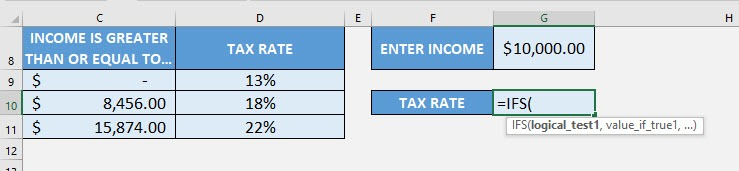STEP 2: The IFS arguments:

## logical_test1, value_if_true1

What is the first condition and value to return if the condition is met?

Let us start from the minimum value of the tax table. If the income is less than \$8456, then the tax rate is 13%

## =IFS(G8<8456, 13%,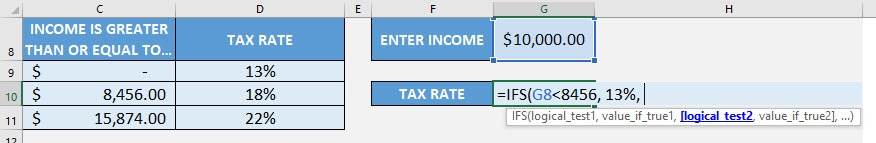## logical_test2, value_if_true2

What is the second condition and value to return if the condition is met?

Going to the second row, if the income is less than \$15874, then the tax rate is 18%

## =IFS(G8<8456, 13%, G8<15874, 18%,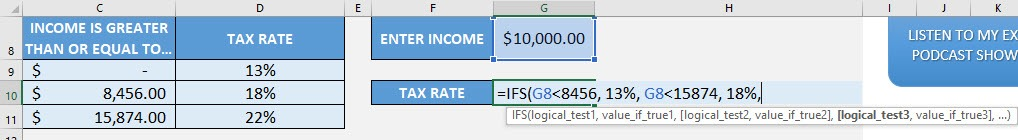## logical_test3, value_if_true3

What is the third condition and value to return if the condition is met?

Going to the last row, if the income is greater than or equal to \$15874, then the tax rate is 22%

## =IFS(G8<8456, 13%, G8<15874, 18%, G8>=15874, 22%)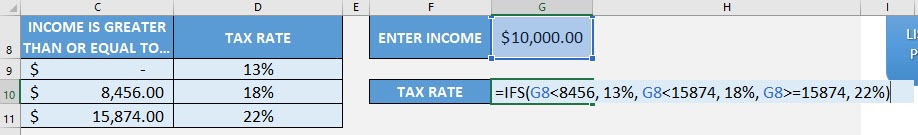You now have your correct tax rate!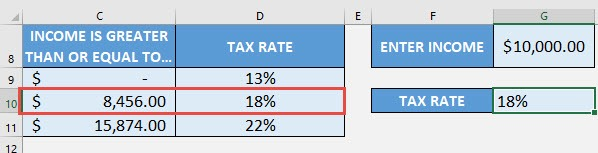How to Use the IFS Formula in Excel